## ML Aggarwal Class 6 Solutions for ICSE Maths Chapter 3 Integers Ex 3.1

Question 1.
Write the opposite of the following:
(i) Loss of ₹ 5000
(ii) 30 km East of Delhi
(iii) 200 m above sea level
(iv) 325 BC
(v) Spending ₹ 2700
(vi) 25°C above freezing point.
Solution:
(i) Profit of ₹ 5000
(ii) 30 km West of Delhi
(iii) 200 m below sea level
(v) Earning ₹ 2700
(vi) 25°C below freezing point.

Question 2.
Write each of the following using appropriate sign ‘+’ or ‘-‘:
(i) Gain of 3 kg Weight
(ii) Earning ₹ 1340
(iii) 20°C below freezing point
(iv) Loss of ₹ 470
(v) Depositing ₹ 2500 in a bank
(vi) 240 m below sea level
(vii) A jet plane flying at a height of 9320 m.
(viii) 6 m down in the basement of a building.
Solution:
(i) +3 kg weight
(ii) +₹ 1340
(iii) -20°C
(iv) -₹ 470
(v) +₹ 2500
(vi) -240 m
(vii) +9320 m
(viii) -6

Question 3.
In each of the following pairs, which number is to the right of the other on the number line?
(i) 3, 5
(ii) 0, -2
(iii) -3, -5
(iv) 2, -7
Solution:
(i) 5
(ii) 0
(iii) -3
(iv) 2

Question 4.
In each of the following pairs, which number is to the left of the other on the number line?
(i) -3, 0
(ii) 2, -5
(iii) -4, -7
(iv) -10,-16
Solution:
(i) -3
(ii) -5
(iii) -7
(iv) -16

Question 5.
Draw a number line and answer the following questions:
(i) Which integers lie between -9 and -2?
(ii) which is the largest among them?
(iii) Which is the smallest among them?
Solution: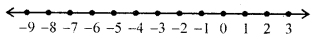(i) -8, -7, -6, -5, -4, -3
(ii) -3
(iii) -8

Question 6.
Write four consecutive integers just greater than -9.
Solution:
The four consecutive integers just than -9 are:
First consecutive = -9 + 1 = -8
Second consecutive = -8 + 1 = -7
Third consecutive = -7 + 1 = -6
Fourth consecutive = -6 + 1 = -5
These are = -8, -7, -6, -5

Question 7.
Write four consecutive integers just before -2.
Solution:
The four consecutive integers just before -2 are:
First consecutive = -2 – 1 = -3
Second consecutive = -3 – 1 = -4
Third consecutive = -4 – 1 = -5
Fourth consecutive = -5 – 1 = -6
∴ These are = -6, -5, -4, -3

Question 8.
Draw a number line and answerthe following questions:
(i) Which number will we reach if we move 6 units to the right of-1?
(ii) Which number will we reach if we move 7 units to the left of 2?
(iii) In which direction should we move to reach 3 from -3?
(iv) In which direction should we move to reach -8 from -3?
Solution:After moving 6 units to the right -1, we reach at 5.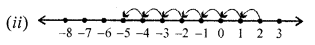After moving 7 units to the left of 2, we reach at -5.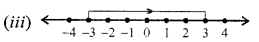To reach 3 from -3, we have to move in right direction.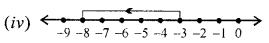To reach -8 from -3, we have to move in left direction.

Question 9.
Using the number line, write the integer which is:
(i) 5 more than -1
(ii) 5 less than -1
(iii) 7 less than 2
(iv) 3 more than -7
Solution:
(i) 5 more than -1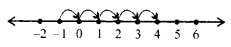Integer 4 is 5 more than -1

(ii) 5 less than -1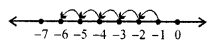Interger -6 is 5 less than -1

(iii) 7 less than 2Interger -5 is 7 less than 2

(iv) 3 more than -7Interger -4 is 3 more than -7

Question 10.
Evaluate the following.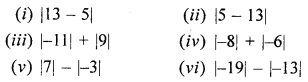Solution: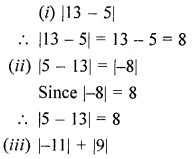Question 11.
Use the appropriate symbol < or > to fill in the following blanks:
(i) -3 ………… 7
(ii) 0 ………….. -2
(iii) -10 ………… -11
(iv) -6 …………….. -2
(v) -5 ………….. -13
(vi) -30 …………… -19
Solution:
(i) -3 < 7
(ii) 0 > -2
(iii) -10 > -11
(iv) -6 < -2
(v) -5 > -13
(vi) -30 < -19

Question 12.
Arrange the following integers in ascending order:
(i) -5, 3, 0, -9, 2
(ii) -28, -33, 9, -4, -31, -2, 35
Solution:
(i) -9, -5, 0, 2, 3
(ii) -33, -31, -28, -4, -2, 9, 35

Question 13.
Arrange the following integers in descending order:
(i) -31,25,-37, 43, 0,-5
(ii) -101, 95, -3, -8, 36, -7, -84
Solution:
(i) 4, 3, 25, 0, -5, -31, -37
(ii) 95, 36, -3, -7, -8, -84, -101

Question 14.
State whether the following statements are true (T) or false (F):
(i) 0 is the smallest positive integer.
(ii) Every negative integer is less than every natural number.
(iii) -7 is to the right of-6 on the number line,
(iv) The absolute value of an integer is always greater than the integer.
Solution:
(i) False
(ii) True
(iii) False
(iv) False